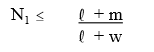# Question: Niebel’s Methods, Standards, & Work Design (13th Edition) –Free Chegg Question Answer

Niebel’s Methods, Standards, & Work Design (13th Edition)

`Transcribed text From Image: `

`Answer:`

### Problem

2. The machining time per piece is 0.164 hours and the machine loading time is 0.038 hours. With an operator rate of \$12.80 per hour and a machine rate of \$14 per hour, calculate the optimum number of machines for lowest cost per unit of output.

### Step-by-step solution

1. Step 1 of 5 As per the stated question, the details are listed below: Machine time per piece = 0.164 hours Machine loading time = 0.038 hours Operator rate = \$12.80 per hour Machine rate = \$14 per hour Walking time to next facility = 0
2. Step 2 of 5 Calculate the optimum number of machines for lowest cost per unit of output. Before that, calculate the number of machines that should be assigned to an operator for lowest cost operations.3. Step 3 of 5 Hence, the number of machines is 5.
4. Step 4 of 5 Now, calculate the optimum number of machines for lowest cost per unit of output by using the formula shown below:Here, K1 = Operator rate K2 = Machine rate l = loading and unloading time m = machine time per piece
5. Step 5 of 5 Hence, assign 5 machines to the operator for lowest cost \$3.35 per piece.